# Technical Test SSC JE: Electrical Engineering (EE)- 5

## 100 Questions MCQ Test Mock Test Series for SSC JE Electrical Engineering | Technical Test SSC JE: Electrical Engineering (EE)- 5

Description
Attempt Technical Test SSC JE: Electrical Engineering (EE)- 5 | 100 questions in 100 minutes | Mock test for Electrical Engineering (EE) preparation | Free important questions MCQ to study Mock Test Series for SSC JE Electrical Engineering for Electrical Engineering (EE) Exam | Download free PDF with solutions
QUESTION: 1

### What is cable tray ?

Solution: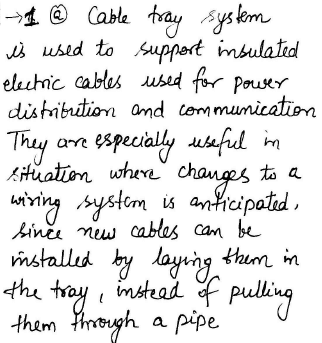QUESTION: 2

Solution:
QUESTION: 3

### In regenerative braking which of the following is true ?

Solution:
QUESTION: 4

The chemical effect of current is used in

Solution:
QUESTION: 5

Unit of deflection sensitivity of a CRO is

Solution:
QUESTION: 6

A 400A, 50 Hz, 30 HP, three phase IM is drawing 50A current at 0.8 p.f. lagging. The stator and rotor copper losses are 1.5 KW and 900 W respectively. The friction andwindage losses are 1050 W and core loss are 1200 W. The air gap power of the motor will be

Solution: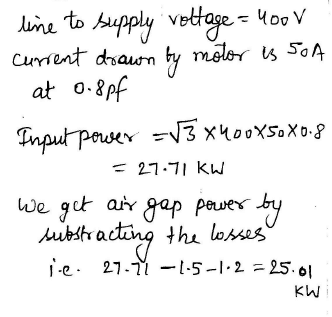QUESTION: 7

The number of armature parallel paths in a two pole D.C. generator having duplex lap winding is

Solution:
QUESTION: 8

The series field of a short shunt D.C. generator is excited by

Solution:
QUESTION: 9

The meter constant of a single phase 230V induction watt hour meter is 400 revolutions per kWh. The speed of the meter disc for a current of 10A of 0.9 pf lagging will be

Solution: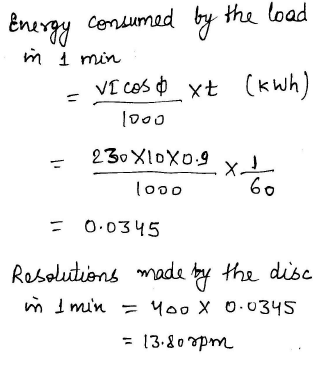QUESTION: 10

Electrostatic voltmeters are particularly suitable for measuring high voltages because the construction is simplified due to

Solution:
QUESTION: 11

A load is connected to an active network. At the terminal to which the load is connected Rn=10W and Vth = 60V. Then the maximum power supplied to the load is

Solution: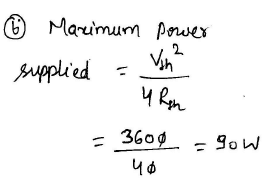QUESTION: 12

The resonant frequency of the given series circuit is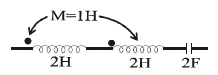Solution: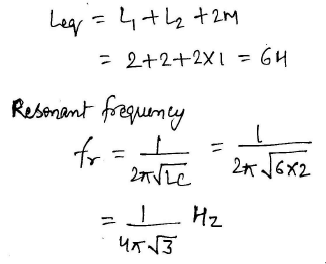QUESTION: 13

What is the correct sequence of the following type of ammeters and voltmeters with increasing accuracy ?

1. Moving iron

2. Moving coil permanent magnet

3. Induction

Select the correct answer using the codes givenbelow

Solution: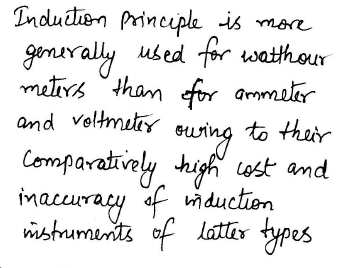QUESTION: 14

Compared with a solid conductor of the same radius, corona appears on a stranded conductor at a lower voltage, because stranding

Solution:
QUESTION: 15

If the separation between the three phase of a transmission line is increased then

Solution:
QUESTION: 16

The mobility of electrons in a material is expressed in units of

Solution:
QUESTION: 17

The impurity commonly used for realizing the base region of a silicon n-p-n transistor is

Solution:
QUESTION: 18

A derive whose characteristics are very close to that of an ideal current source is

Solution: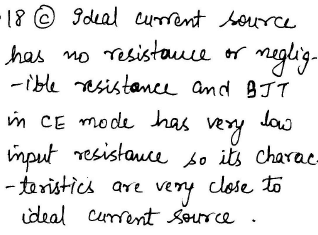QUESTION: 19

A galvanometer can be converted into an ammeter by connecting

Solution: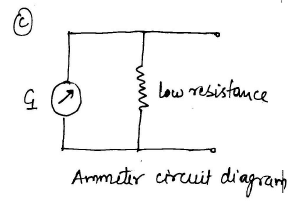QUESTION: 20

The delta connected generator shown in the figure has phase voltage of 200 V on no load.If connection of one of the phase is reversed then resultant voltage across the mesh will be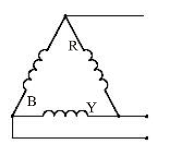Solution: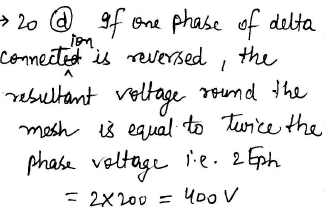QUESTION: 21

Wooden cross arms are used for supporting

Solution:
QUESTION: 22

A moving iron instrument gives full scale deflection with 10 mA has a resistance of 3W. Determine the resistance to be connected in series so as to enable it to read up to 100V

Solution: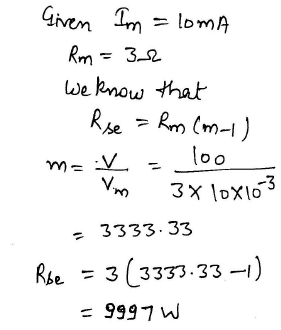QUESTION: 23

The rotor of a single phase induction motor

Solution:
QUESTION: 24

An insulating material ring has mean diameter of 100 mm, cross sectional area of 200 mm2.It is wound with 200 turns of insulated wire. Another coil of 1000 turns is wound on the top of the first coil. Then what is the mutual inductance ?

Solution: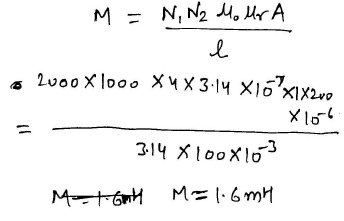QUESTION: 25

In forward mode NPN BJT, if we increase the voltage Vcc, the collector current increases

Solution:
QUESTION: 26

During electric arc welding as the thickness of the metal to be welded increases

Solution:
QUESTION: 27

In fluorescent tube, a ballast resistance is connected in series with the choke

Solution:
QUESTION: 28

The current through 120 ohm resistor in the circuit shown in the figure below is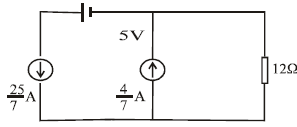Solution: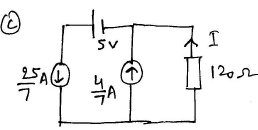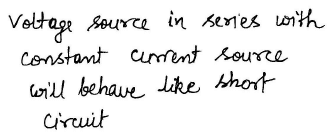QUESTION: 29

For the circuit shown below, what is the voltage across the current source Is ?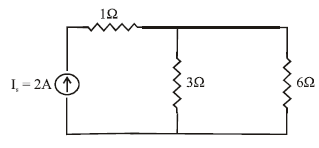Solution: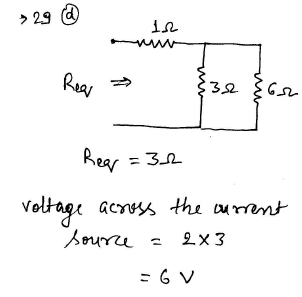QUESTION: 30

To determine the losses of an alternator, it is driven by

Solution:QUESTION: 31

A 300 kW alternator is driven by a prime mover of speed regulation 4% while the prime mover of another 200 kW alternator has a speed regulation of 3%. When operating in parallel,the total load they can take without any of them being overloaded is

Solution: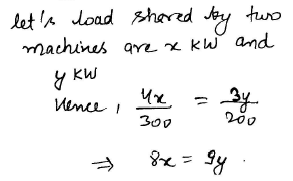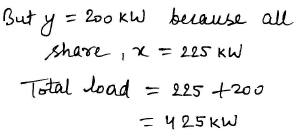QUESTION: 32

A voltage source having an internal impedance of 8 + j6W supplies power to resistive load.What should be the load resistance for maximum power transferred to it ?

Solution: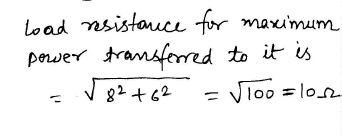QUESTION: 33

The power delivered to a 3-phase load can be measured by the use of 2 wattmeters only when the

Solution:
QUESTION: 34

The steady state current in the R-C series circuit, on the application of step voltage of magnitude E will be

Solution:
QUESTION: 35

CE configuration is the most preferred transistor configuration when used as a switch because it

Solution: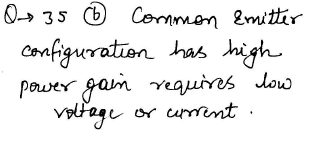QUESTION: 36

Silicon devices are preferred at high temperature operations in comparison to germanium because

Solution:
QUESTION: 37

Which of the following specification is not correct for a common collector amplifier ?

Solution:
QUESTION: 38

If R, L and C are the parameters of resistors. What is the condition for the resistor to benon-inductive ?

Solution:
QUESTION: 39

The frequency response shown in the figure corresponds to a

Solution:
QUESTION: 40

A 100 kV, 50 Hz supply is fed to a rectifier ammeter (using a bridge rectifier) through a capacitor. The PMMC ammeter of the rectifier instrument reads 45×10-3 Amp. What is the value of the capacitor ?

Solution: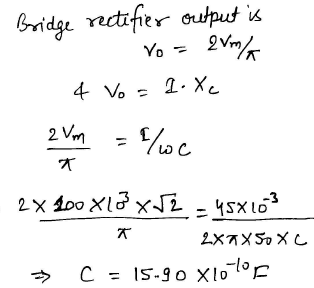QUESTION: 41

The full load copper loss and iron loss of a transformer are 6400 W and 5000 W respectively. The copper loss and iron loss at half load will be respectively

Solution: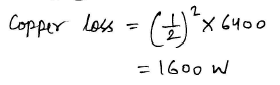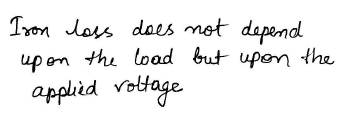QUESTION: 42

Normally which of the following is used, when a large scale conversion from AC to DC power is required ?

Solution:
QUESTION: 43

The purpose of providing iron-core in a step up transformer is

Solution:
QUESTION: 44

Two p-n junction diodes connected back to back to make a transistor which of the following is correct ?

Solution: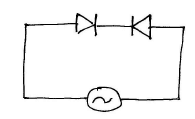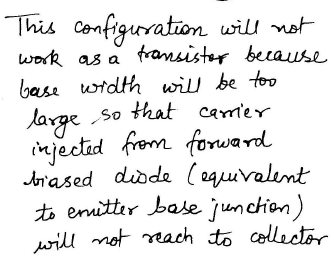QUESTION: 45

A 240 V, dc shunt motor takes a total current of 24 amp. Resistance of shunt field winding is 120W and armature has 0.3W. What is the current in the armature ?

Solution: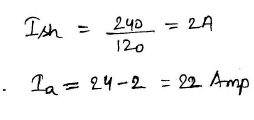QUESTION: 46

The torque characteristics of a 3-phase induction motor is similar to that of a

Solution:
QUESTION: 47

For what value of unknown resistance x, the potential difference between B and D will bezero in the circuit shown in figure?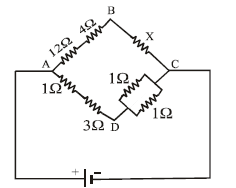Solution: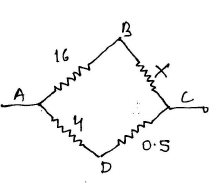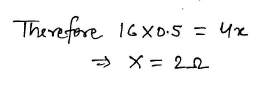QUESTION: 48

Method of speed control used on 25 kV, 50 Hz single phase traction is same as

Solution: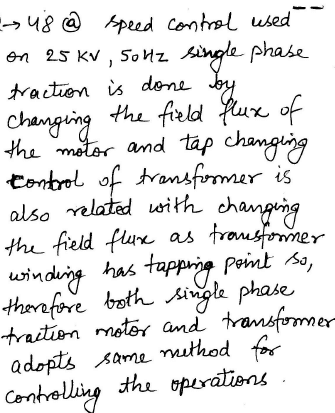QUESTION: 49

Which motor is a variable speed motor ?

Solution:
QUESTION: 50

For a given emitter current the collector current can be increased by

Solution:
QUESTION: 51

What is the value of current I4 (in A) for the given junction?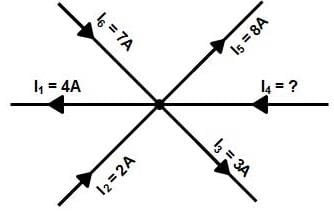Solution:

According to Kirchhoff’s Current Law: At any point in an electrical circuit, the sum of currents flowing towards that point is equal to the sum of currents flowing away from that point.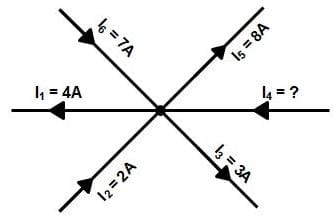From the above Diagram
Current Flowing towards the Point: I2, I6, I4
Current Flowing Away from the Point: I1, I3, I5
Hence I2 + I6 + I4 = I1 + I3 + I5
Putting the value of the current
2A + 7A + I4 = 4A + 3A + 8A
I4 = 15A − 9A = 6A
I4 = 6A

QUESTION: 52

Cooling of synchronous generator is done by

Solution:
QUESTION: 53

In dc shunt motor, regenerative braking is limited by

Solution:
QUESTION: 54

The least significant feature while selecting a motor for centrifugal pump is

Solution:
QUESTION: 55

Lightening arresters are used in power system to protect electrical equipment against

Solution:
QUESTION: 56

For protection of rotating machines against lightening surges _____ is used

Solution:
QUESTION: 57

An inductor with a ferromagnetic core is supplied from a sinusoidal voltage source with frequency 'f'. The current drawn by the inductor will be

Solution:
QUESTION: 58

Which one of the following is a speed control method of three phase squirrel cage induction motor

Solution:
QUESTION: 59

To maximize the driving torque in an induction type instrument, the flux produced by shunt coil and series coil should be

Solution:
QUESTION: 60

Which one among the following has the highest numerical value in a stepper motor?

Solution:
QUESTION: 61

The motor which can produce uniform torque from standstill to synchronous speed is

Solution:
QUESTION: 62

A voltage source having an open circuit voltage of 120 V and internal resistance of 60W, is equivalent to a current source of

Solution:QUESTION: 63

Purely inductive current takes power from the ac mains when

Solution:
QUESTION: 64

A square wave is fed to an R-C circuit. Then

Solution:
QUESTION: 65

To improve pf in three phase circuits, the capacitor bank is connected in delta to make

Solution:
QUESTION: 66

A frog leg winding is used in dc machine to eliminate

Solution:
QUESTION: 67

In dc machine armature winding, the no. of commutator segments is equal to the number of armature

Solution:
QUESTION: 68

A cumulatively compounded dc generator is supplying 20A at 200V. Now if the series field winding is short circuited, the terminal voltage

Solution:
QUESTION: 69

Stable operation of an induction motor is

Solution:
QUESTION: 70

In a 3-f induction motor iron loss mainly occurs in

Solution:
QUESTION: 71

For under frequency operation the eddy current loss

Solution:
QUESTION: 72

A power system needs injection of VARs at

Solution:
QUESTION: 73

A thermal generating station has an installed capacity of 15 MW and supplies a daily load of 10 MW for 12 hours and 5 MW of remaining 12 hours. The plant capacity factor for this station is

Solution: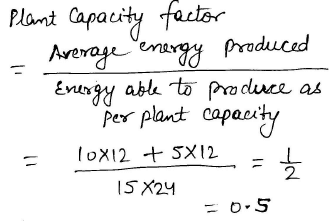QUESTION: 74

The material used for the manufacture of ground wire is

Solution:
QUESTION: 75

A PIN diode is frequently used as a

Solution:
QUESTION: 76

ICBO in a transistor can be reduced by reducing

Solution:
QUESTION: 77

Avalanche breakdown is primarily dependent on the phenomenon of

Solution:
QUESTION: 78

In case of GLS lamps the increase in supply voltage reduces

Solution:
QUESTION: 79

The illumination at various points on a horizontal surface illuminated by same source varies as

Solution:
QUESTION: 80

In a purely resistive circuit, the average power is ____ the peak power Pmax

Solution:
QUESTION: 81

When a transformer is operating on no load, the primary applied voltage is approximately balanced by

Solution:
QUESTION: 82

The inductive reactance of a transformer depends on

Solution:
QUESTION: 83

Constant current transformer is of ____ type

Solution:
QUESTION: 84

The emf generated due to nth harmonic component of flux in an alternator will be____the fundamental emf in magnitude

Solution:
QUESTION: 85

The armature reaction effect in synchronous machine depends on

Solution:
QUESTION: 86

Aluminium conductor cables can be joined by

Solution:
QUESTION: 87

The permissible variation of frequency in power system is

Solution:
QUESTION: 88

Generators for power plant to supply exclusively peak loads are usually designed for maximum efficiency to occur at

Solution:
QUESTION: 89

Two part tariff is charged on the basis of

Solution:
QUESTION: 90

An industrial installation has a power factor of 0.8 lagging. It would be economical to improve pf to

Solution:
QUESTION: 91

The charging current in a transmission line increases due to corona effect because corona increases

Solution:
QUESTION: 92

For 11 kv line, the inductance per km per phase will be of the order of

Solution:
QUESTION: 93

As compared to sending and voltage the receiving end voltage of a short line under no load condition is

Solution:
QUESTION: 94

In 3-phase, 4-wire cable, the x-sectional area of neutral conductor is

Solution:

The 3-Phase four wire system is used for the distribution system. A three-phase four-wire supply gives a consumer the choice of a 400 V three-phase supply and a 230 V single-phase supply. Many industrial loads such as motors require a three-phase 400 V supply, while the lighting load in a factory, as in a house, will be 230 V. Industrial loads usually demand more power than a domestic load, and more power can be supplied by a 400 V three-phase supply than is possible with a 230 V single-phase supply for a given size of cable since power = VI cosφ (watts).

This form of connection is shown in Fig. and is known as a star or Y connection. With the resulting 4-wire system, the three loads also are connected in star configuration The three outer wires are called the lines, and the common wire in the center is called the neutral. One reason for the connection of the neutral conductor is to provide a path for currents if the system became unbalanced. Another is that it enables single-phase loads to be connected to a three-phase system.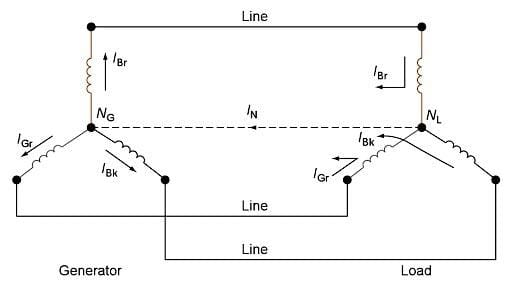The addition of the conductor between the star points converts the system into what is known as a ‘three-phase four-wire system‘. We can see that the currents supplied by the generator flow along the lines, through the load and return via the neutral conductor.

However, in a balanced three-phase system are equal, and add up to the neutral current, which is zero:

IBr + IBk + IGr = IN = 0

If the three loads were identical in every way (same impedance and phase angle), then the currents flowing in the three lines would be identical. The three currents meet at the star point of the load. The resultant current returning down the neutral wire would, therefore, be zero. The load, in this case, is known as a balanced load, and the neutral is not strictly necessary. However, it is difficult, in practice, to ensure that each of the three loads is exactly balanced. For this reason, the neutral is left in place. Also, since it has to carry only the relatively small ‘out-of-balance’ current, it is made half the cross-sectional area of the lines.

In some cases, the same-sized cable is normally used for all four wires both to allow for inevitable imbalances and to also ensure that the neutral cable has equal tensile strength as the phase conductors.

QUESTION: 95

The insulation resistance of a single core cable is 200 MW/km. The insulation resistance for 5km length is

Solution:
QUESTION: 96

The magnitude of fault current depends upon

Solution:
QUESTION: 97

For a fault at the terminals of synchronous generator, the fault current is maximum for a

Solution:
QUESTION: 98

Zero sequence current can flow from a line to transformer bank if the windings are in

Solution:
QUESTION: 99

A dc series motor must not be started on no load because it would

Solution:
QUESTION: 100

A dc shunt motor may have rising speed torque characteristics due to

Solution:Use Code STAYHOME200 and get INR 200 additional OFF Use Coupon Code